Function Repository Resource:

# EinsteinSolid

Make a simulation of the Einstein solid

Contributed by: Enrique Zeleny
 ResourceFunction["EinsteinSolid"][array,steps] makes a simulation of the Einstein solid starting with array and using steps iterations.

## Details and Options

At low temperatures, the Dulong–Petit law fails and the specific heat capacity at constant volume CV of solids is no longer constant. The Einstein quantum model for a solid correctly explains this phenomenon. We can make a simplified model of a solid with a 2D cell array (Black and Ogborn, 1976) with one oscillator in each cell with an energy of a multiple of ω, and thermal interaction reproduced through a transfer of a quantum of energy between a pair of these cells chosen randomly.

## Examples

### Basic Examples (2)

The initial state assigns one quantum to every cell. After about 1,000 steps, the distribution of a thermal equilibrium state and Boltzmann distribution is obtained; it is stable after more steps:

 In:=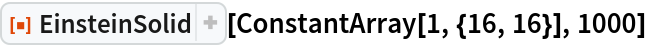Out=Plot the results:

 In:=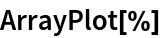Out=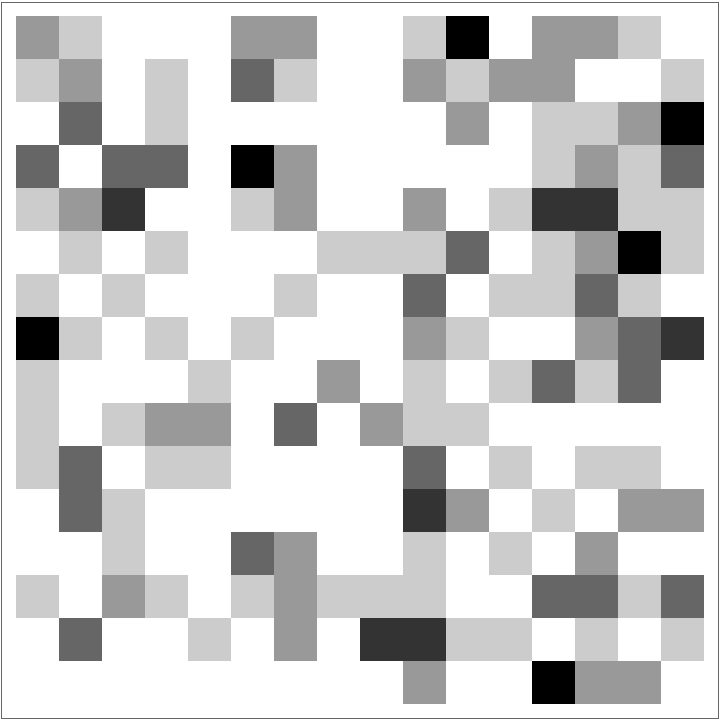In:=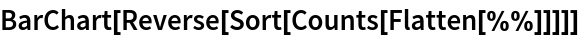Out=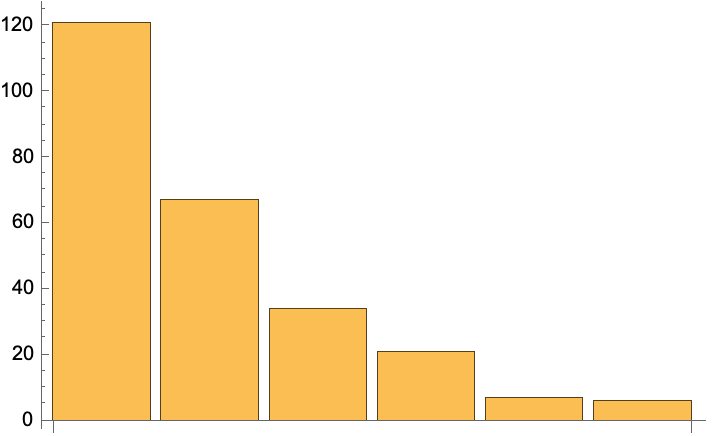After about 20,000 steps, the distribution of energies closely approximates the Boltzmann distribution, and thermal equilibrium is obtained:

 In:=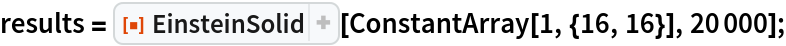In:=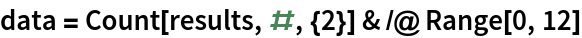Out=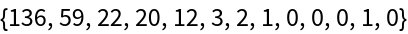The left chart represents instantaneous values m(ε), the right chart represents the mean values given by the canonical distribution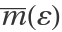(equal to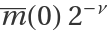) and the red curve represents the fit of the occupancies:

 In:=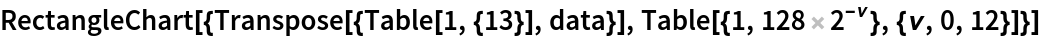Out=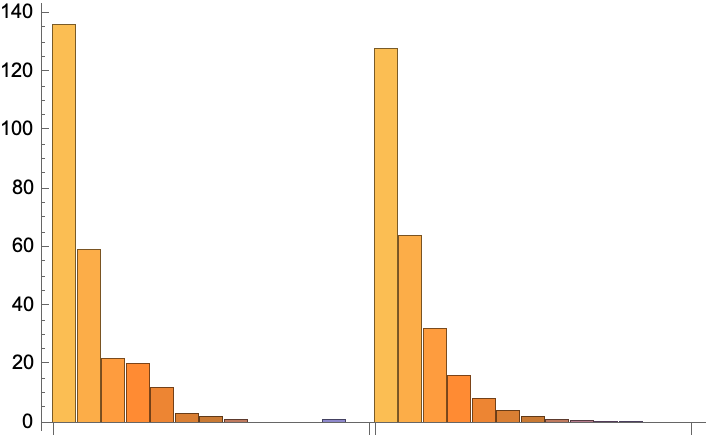Fitting data:

 In:=Out=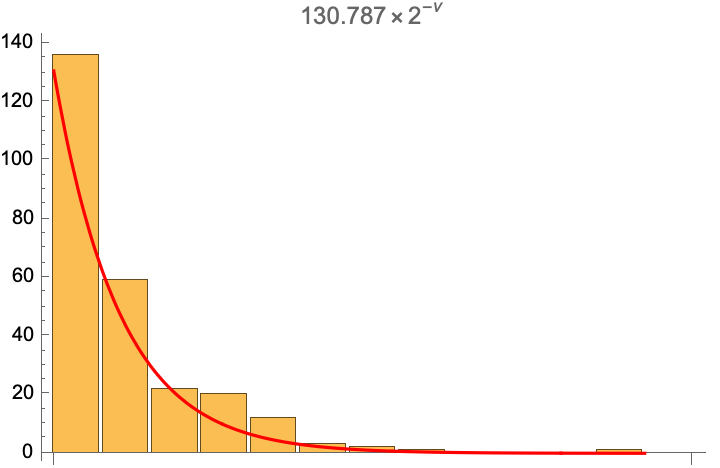The number of points assigned to each cell is the number of quanta possessed by the oscillator (occupancy):

 In:=Out=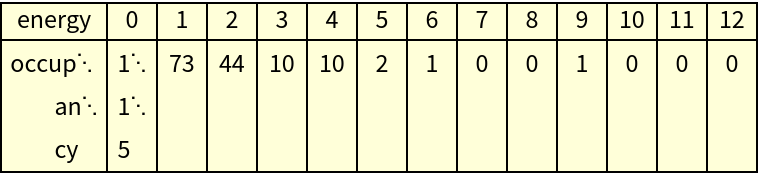## Requirements

Wolfram Language 11.3 (March 2018) or above

## Version History

• 1.0.0 – 25 February 2019